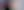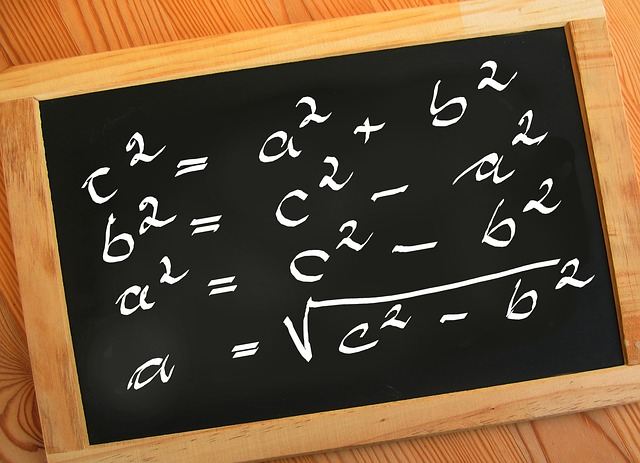GMAT Test PracticeGMAT Data Sufficiency

Updated December 14, 2021

GMAT Test Practice

Data sufficiency questions sit alongside Problem-Solving in the Quantitative Reasoning section of the GMAT. They test a candidate’s ability to critically evaluate data, and decide whether or not there is sufficient information for a specific problem to be solved.

Questions of this kind require individuals to draw on their skills in algebra, arithmetic, data interpretation, applied math and geometry.

The Quantitative section carries significant weight in GMAT scoring. The section is scored on a scale of 60 (in intervals of one). The total test score is reported from the Verbal and Quantitative sections combined.

The GMAT, including the Data Sufficiency section, is a computer-adaptive test, which means that the system reacts to your ability. If you get a question wrong, you will be given easier questions; if you get the easier questions correct, you will be given more difficult questions. The harder the question, the higher the score value.What to Expect From Data Sufficiency Questions

Data Sufficiency questions all follow the same pattern: a question, followed by two statements.

The task is to work out whether:

• One or both of the statements alone is/are sufficient for the question to be answered
• The statements in isolation are useless but together they can solve the problem
• Neither statement is of any use and the problem cannot be solved

The list of answer options are presented from A to E and are always the same.

For example:

Problem:

Is X > 9

Statements:

(1) X > 8
(2) X > 10

(A) Statement 1 alone is sufficient, but statement 2 alone is not sufficient
(B) Statement 2 alone is sufficient, but statement 1 alone is not sufficient
(C) Both statements together are sufficient, but neither statement alone is sufficient
(D) Each statement alone is sufficient
(E) Statements 1 and 2 together are not sufficient

The challenge is not to answer the actual question, but rather to decide whether or not you have enough information to be able to answer it.

There are 31 questions in the Quantitative Reasoning section of the GMAT and between a quarter and a third of these will be Data Sufficiency questions.

You will have 75 minutes to answer a total of 37 questions (you won’t know how many of those questions will be Problem-Solving and how many will be Data Sufficiency until you see the test paper).

Key Tips for GMAT Data Sufficiency Questions

The answer statements are the same for every question in a Data Sufficiency question:

(A) Statement 1 alone is sufficient, but statement 2 alone is not sufficient
(B) Statement 2 alone is sufficient, but statement 1 alone is not sufficient
(C) Both statements together are sufficient, but neither statement alone is sufficient
(D) Each statement alone is sufficient
(E) Statements 1 and 2 together are not sufficient

You should allocate only around two minutes to answering each Data Sufficiency question, so you won’t want to waste time worrying about the answer options during the test. Ensure you know these off by heart and understand what each means before you sit the test.

Memorizing these statements will give you a better chance of being able to quickly work with the data provided to find the correct answer option.

2. Use a Process of Elimination – Take Each Statement in Isolation

The most reliable way of determining the correct answer is to tackle each statement in isolation. It is usually best to choose the statement that looks the easiest to understand first; you don’t have to tackle them in order.

• If statement 1 is insufficient, you can quickly eliminate answer options A and D

• If statement 2 is insufficient, you can quickly eliminate answer options B and D

• If the first statement you look at is sufficient, you can quickly check if the second is also sufficient (answer option D)

• After this, all you will need to ascertain is whether the statements become sufficient when they are used together (answer option C) or whether they are still insufficient (answer option E).

3. You Don’t Need to Know the Answer

It’s easy to get so engrossed in the problem that you find yourself trying to work out the answer. Keep in mind at all times that you should be working through the statements, eliminating the answers which do not apply, finding the one that does and moving on to the next question.

Often candidates get stuck trying to work out what ‘x’ is and, in many cases, they don’t need to know.

4. Remember, the Answer Might Not Be a Whole Number

It’s often useful to plug in a real number for the variables in the equations to make things clearer as you work through the statements. However, it pays to remember that those variables could be anything – a positive or negative decimal, a negative integer, a positive fraction, a negative fraction, etc.

If a statement says x > 8, you might think that x must be 9 or above, when actually it could be anywhere in the infinity of decimals between two whole numbers. Keep in mind that the mystery number could be absolutely anything, not just a simple, neat number.

5. Revise the Basics

Data Sufficiency questions are based around basic math concepts that you should have covered at school. Make sure you brush up on your multiplication and division, especially when it comes to fractions and decimals, and that you understand the basics of working with equations.

Calculators are not permitted on the GMAT, so it's advisable to practice your mental arithmetic before your test.

However, make sure you remember that these are not math tests. You will do well on your Data Sufficiency questions if you utilize skills like estimation, problem-solving and reasoning.Example Data Sufficiency Questions

Now you know what to expect when faced with Data Sufficiency questions, below are three examples to help you practice.

Example Question 1

What is the total cost of 10 identical cupboards and 10 identical drawers?

(1) 6 cupboards and 4 drawers cost a total of \$390
(2) 4 cupboards and 6 drawers cost a total of \$510

(A) Statement 1 alone is sufficient, but statement 2 alone is not sufficient
(B) Statement 2 alone is sufficient, but statement 1 alone is not sufficient
(C) Both statements together are sufficient, but neither statement alone is sufficient
(D) Each statement alone is sufficient
(E) Statements 1 and 2 together are not sufficient

Start by taking statement (1) in isolation. An equation for the information you have is:

6C + 4D = \$390

This equation has two variables and therefore cannot be solved; statement (1) is, therefore, insufficient to answer the question, so you can rule out answers A and D.

Next, take statement (2). An equation representing this statement is:

4C + 6D = \$510

Again, this is a linear equation with two variables and therefore it cannot be solved. Knowing this, we can now eliminate answer B.

Both statements now need to be considered together.

You now have two equations with the same variables. When placed together, these two equations can be solved to provide values for ‘C’ and ‘D’.

This is the point at which you need to remember to stop; you don’t need to solve either equation. You already have your answer, C.Example Question 2

If ‘n’ is a member of the set 16, 19, 21, 22, 24, 27, 36, 42 what is the value of ‘n’?

(1) ‘n’ is even
(2) ‘n’ is a multiple of 3

(A) Statement 1 alone is sufficient, but statement 2 alone is not sufficient

(B) Statement 2 alone is sufficient, but statement 1 alone is not sufficient

(C) Both statements together are sufficient, but neither statement alone is sufficient

(D) Each statement alone is sufficient

(E) Statements 1 and 2 together are not sufficient

The question is asking us to ascertain the value of ‘n’. Therefore, if the statements are to be sufficient, either or both must reveal which of the numbers ‘n’ is.

Statement (1) states that ‘n’ is even, which means it can be 16, 22, 24, 36 or 42. That’s as far as we can get with statement (1), so we know that statement (1) is not sufficient, ruling out answers A and D.

Statement (2) suggests that ‘n’ could be 21, 27, 36 or 42. There is, however, no further information to help us decide which. Statement (2) is therefore not sufficient, ruling out B.

When it comes to combining the statements, we know that ‘n’ is even and is a multiple of three; this leaves us with the possibility of ‘n’ being 36 or 42. However, we have no way of determining which of these two numbers is ‘n’.

Therefore, even together, the statements are not sufficient, ruling out C, so the correct answer is E.

Example Question 3

A supermarket chain agreed to donate ‘x’ dollars to a homeless charity for every loaf of bread its stores sold during a Help the Homeless week. What was the total amount expected to be donated?

(1) A total of 5,000 loaves were expected to be sold

(2) 120 more loaves were sold than expected, so the total amount actually donated was \$2,500

(A) Statement 1 alone is sufficient, but statement 2 alone is not sufficient

(B) Statement 2 alone is sufficient, but statement 1 alone is not sufficient
(C) Both statements together are sufficient, but neither statement alone is sufficient

(D) Each statement alone is sufficient

(E) Statements 1 and 2 together are not sufficient

Start with the first statement. We know that 5,000 loaves of bread were expected to be sold and ‘x’ dollars will be donated for each one. The expected donation is, therefore:

5,000x

However, we can’t work out the value of ‘x’ from statement (1) which means we can eliminate answers A and D.

Next, statement (2); we are told that 120 more loaves were sold than expected. Therefore, the amount donated for these extra loaves could be worked out using the equation:

120x

We also find out that the total amount donated was \$2,500. As the question is asking for the total amount expected (rather than the total amount received) we need to take the extra amount donated from the total:

\$2,500 – 120x

However, we still do not know the value of ‘x’, so we can now rule out answer option B.

Having dealt with each statement individually, we can now put them together and can see that this equation can be solved:

5,000x = \$2,500 – 120x

Remember – this is as far as you go. You don’t need to solve the equation, you just need to know that it can be worked out with both statements, so the correct answer is: C.

Final Thoughts

There are three ways in which you can attempt to find the answer to a Data Sufficiency question: you can use algebra, make an informed guess or plug in numbers (assign values to the variables) to help you work out whether or not the statements are sufficient­­. Which method you use will depend on the nature of the question.

However you tackle the questions, remember to stop as soon as you have established the A to E answer, rather than the actual answer to the question.

As well as memorizing the A to E list of options, it pays to also memorize the fact that, in every instance, once you have analyzed statement (1) you can eliminate either A and D or B, C and E. The quicker you can narrow down the options, the more questions you will be able to get through.